# PSAT Math : Integers

## Example Questions

### Example Question #1 : How To Multiply Even Numbers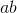is even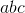is even

Therefore, which of the following must be true about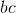?

It must be even.

It could be either even or odd.

It must be odd.

It could be either even or odd.

Explanation:

Recall that when you multiply by an even number, you get an even product.

Therefore, we know the following from the first statement:is even oris even or bothandare even.

For the second, we know this:

Sinceis even, therefore,can be either even or odd. (Regardless of what it is, we can get an even value for.)

Based on all this data, we can tell nothing necessarily about. Ifis even, thenis even, even ifis odd. However, ifis odd whileis even, thenwill be even.

### Example Question #31 : Even / Odd Numbers

In a group of philosophers,are followers of Durandus. Twice that number are followers of Ockham. Four times the number of followers of Ockham are followers of Aquinas. One sixth of the number of followers of Aquinas are followers of Scotus. How many total philosophers are in the group?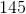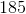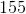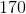Explanation:

In a group of philosophers,are followers of Durandus. Twice that number are followers of Ockham. Four times the number of followers of Ockham are followers of Aquinas. One sixth of the number of followers of Aquinas are followers of Scotus. How many total philosophers are in the group?

To start, let's calculate the total philosophers:

Ockham:* <Number following Durandus>, or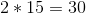Aquinas:* <Number following Ockham>, or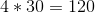Scotus:divided by, orTherefore, the total number is: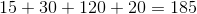### Example Question #1 : How To Multiply Even Numbers

If n is an integer that is not equal to 0, which of the following must be greater than or equal to n?

I. 7n

II. n + 5

III. n2

II and III only

I, II, and III

II only

I and III only

I and II only

II and III only

Explanation:

I is not always true because a negative number multiplied by 7 will give a number that is more negative than the original. II is true because adding 5 to any number will increase the value. III is true because squaring any number will increase the magnitude of the value, and squaring a negative number will make it positive.

### Example Question #1 : How To Multiply Even Numbers

Which of the following integers has an even integer value for all positive integers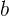and?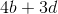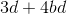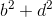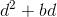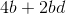Explanation:

There are certain patterns that can be used to predict whether the product or sum of numbers will be odd or even. The sum of two odd numbers is always even, as is the sum of two even numbers. The sum of an odd number and an even number is always odd. In multiplication the product of two odd numbers is always odd. While the product of even numbers, as well as the product of odd numbers multiplied by even numbers is always even. So for this problem we need to find scenarios where the only possibile answers are even.can only result in even numbers no matter what positive integers are used forand, because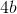must can only result in even products; the same can be said for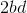. The rules provide that the sum of two even numbers is even, sois the answer.

### Example Question #1 : How To Find The Least Common Multiple

How many positive integers less than ten thousand are multiples of both eight and eighteen?

139

72

70

138

555

138

Explanation:

In order to find all of the numbers that are multiples of both 8 and 18, we need to find the least common mutliple (LCM) of 8 and 18. The easiest way to do this would be to list out the multiples of 8 and 18 and determine the smallest one that is common to both.

First, let's list the first several multiples of eight:

8, 16, 24, 32, 40, 48, 56, 64, 72, 80, 88 . . .

Next, we list the first several multiples of eighteen:

18, 36, 54, 72, 90, 108, 126, 144 . . .

By comparing the multiples of eight and eighteen, we can see that the smallest one that they share is 72. Thus, the LCM of 8 and 18 is 72.

Because the LCM is 72, this means that every multiple of 72 is also a multiple of both 8 and 18. So, in order to find all of the multiples less than ten thousand that are both multiples of 8 and 18, we simply need to find how many multiples of 72 are less than 10000, and to do this, all we have to do is to divide 10000 by 72.

When we divide 10000 by 72, we get 138 with a remainder of 64; therefore, 72 will go into ten thousand 138 times before it exceeds ten thousand. In other words, there are 138 numbers less than 10000 that are multiples of 72 and, by extension, also multiples of both 8 and 18.

### Example Question #1 : Factors / Multiples

Ifis divisible by 2, 4 and 7, which of the following is a possible value of?

114

172

154

136

168

168

Explanation:

The simplest way to solve this question is to go through each answer choice and check to see if it is divisible by 2, 4 and 7. When we do this, we find that only 168 is evenly divisible by each of these numbers. 114 is not divisible by 4 or 7, 172 is not divisibly by 7, 136 is not divisble by 7, and 154 is not divisible by 4.

### Example Question #1 : Factors / Multiples

If a, b, and c are positive integers such that 4a = 6b = 11c, then what is the smallest possible value of c?

67

132

33

121

11

67

Explanation:

We are told that a, b, and c are integers, and that 4a = 6b = 11c. Because a, b, and c are positive integers, this means that 4a represents all of the multiples of 4, 6b represents the multiples of 6, and 11c represents the multiples of 11. Essentially, we will need to find the least common multiples (LCM) of 4, 6, and 11, so that 4a, 6b, and 11c are all equal to one another.

First, let's find the LCM of 4 and 6. We can list the multiples of each, and determine the smallest multiple they have in common. The multiples of 4 and 6 are as follows:

4: 4, 8, 12, 16, 20, ...

6: 6, 12, 18, 24, 30, ...

The smallest multiple that 4 and 6 have in common is 12. Thus, the LCM of 4 and 6 is 12.

We must now find the LCM of 12 and 11, because we know that any multiple of 12 will also be a multiple of 4 and 6.

Let's list the first several multiples of 12 and 11:

12: 12, 24, 36, 48, 60, 72, 84, 96, 108, 120, 132, ...

11: 11, 22, 33, 44, 55, 66, 77, 88, 99, 110, 121, 132, ...

The LCM of 12 and 11 is 132.

Thus, the LCM of 4, 6, and 12 is 132.

Now, we need to find the values of a, b, and c, such that 4a = 6b = 12c = 132.

4a = 132

Divide each side by 4.

a = 33

Next, let 6b = 132.

6b = 132

Divide both sides by 6.

b = 22

Finally, let 11c = 132.

11c = 132

Divide both sides by 11.

c = 12.

Thus, a = 33, b = 22, and c = 12.

We are asked to find the value of a + b + c.

33 + 22 + 12 = 67.

### Example Question #64 : Integers

If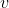is divisible by 2, 3 and 15, which of the following is also divisible by these numbers?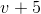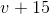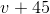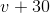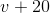Explanation:

Since v is divisible by 2, 3 and 15, v must be a multiple of 30. Any number that is divisible by both 2 and 15 must be divisible by their product, 30, since this is the least common multiple.

Out of all the answer choices, v + 30 is the only one that equals a multiple of 30.

### Example Question #711 : Arithmetic

Suppose thatis an integer such that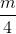is ten greater than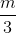. What is the value of?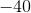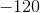Explanation:

We are given information that m/4 is 10 greater than m/3. We set up an equation where m/4 = m/3 + 10.

We must then give the m variables a common denominator in order to solve for m. Since 3 * 4 = 12, we can use 12 as our denominator for both m variables.

m/4 = m/3 + 10 (Multiply m/4 by 3 in the numerator and denominator.)

3m/12 = m/3 + 10 (Multiply m/3 by 4 in the numerator and denominator.)

3m/12 = 4m/12 + 10 (Subtract 4m/12 on both sides.)

-m/12 = 10 (Multiply both sides by -12.)

m = -120

-120/4 = -30 and -120/3 = -40. -30 is 10 greater than -40.

### Example Question #1 : How To Find The Greatest Common Factor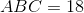, andare distinct integers.

Which of the following is a possible value of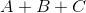?Explanation:

We need to find ways to factor 18 such that the three factors are different, and then find the sum of those factors in each case.

18 can be factored as the product of three integers in four ways:

I)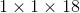II)III)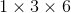IV)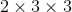Disregard I and IV since each repeats a factor.

In (II), the sum of the factors is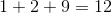; in (III) the sum is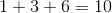. Of the five choices, only 12 is possible.

### All PSAT Math Resources